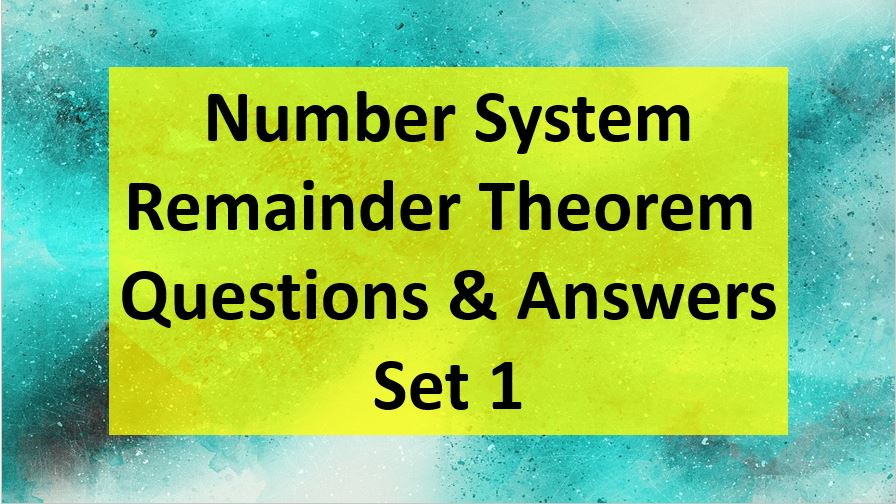Monday, September 27, 2021
Home > Question and Answer > Remainder Theorem Question and Answer Set 1

# Remainder Theorem Question and Answer Set 1# Remainder Theorem Question and Answer Set 1

Hi students, welcome to Amans Maths Blogs (AMB). On this post, you will get the Remainder Theorem Question and Answer Set 1 is the questions with solution for SSC CGL CHSL CAT and other competative exams like NTSE NSEJS NMTC etc. It will help you to practice the questions on the topics of maths as Remainder Theorem.

Remainder Theorem Question and Answer Set 1: Ques No 1

Find the remainder when 84 + 98+ 197 + 240 + 140  is divided by 32

Options:

A. 19

B. 24

C. 23

D. 13

Remainder Theorem Question and Answer Set 1: Ques No 2

Find the reminder when 1! + 2! + 3! + . . . . .. . .. 99! + 100! is divided by 7!.

Options:

A. 873

B. 824

C. 723

D. 613

Remainder Theorem Question and Answer Set 1: Ques No 3

Find the reminder when 1! + 2! + 3! + . . . . .. . .. 99! + 100! is divided by 7!.

Options:

A. 873

B. 824

C. 723

D. 613

Remainder Theorem Question and Answer Set 1: Ques No 4

What is the remainder when 1318 is divided by 19?

Options:

A. 13

B. 14

C. 3

D. 1

Remainder Theorem Questions and Answer Set 1: Ques No 5

What is the remainder when 123 × 124 × 125 is divided by 9.

Options:

A. 1

B. 3

C. 5

D. 7

Remainder Theorem Questions and Answer Set 1: Ques No 6

What is the remainder of 192200002 divided by 23?

Options:

A. 16

B. 13

C. 15

D. 17

Remainder Theorem Questions and Answer Set 1: Ques No 7

What is the remainder of (321)5687 divided by 8?

Options:

A. 2

B. 1

C. 5

D. 7

Remainder Theorem Questions and Answer Set 1: Ques No 8

What is the remainder of 1421 x 1423 x 1425 divided by 12?

Options:

A. 2

B. 5

C. 3

D. 8

Remainder Theorem Questions and Answer Set 1: Ques No 9

What is the remainder of (146)56 divided by 7?

Options:

A. 4

B. 3

C. 5

D. 1

Remainder Theorem Questions and Answer Set 1: Ques No 10

What is the remainder when 1! + 2! + 3! + … + 99! + 100! divided by 1x2x3x4x5x6x7?

Options:

A. 837

B. 873

C. 623

D. 725

Remainder Theorem Questions and Answer Set 1: Ques No 11

What is the remainder when (269)57587 divided by 6?

Options:

A. 5

B. 8

C. 6

D. 7

Remainder Theorem Questions and Answer Set 1: Ques No 12

What is the remainder when (64)999 divided by 7?

Options:

A. 3

B. 5

C. 1

D. 7

Remainder Theorem Questions and Answer Set 1: Ques No 13

What is the remainder when 73 x 75 x 78 x 57 x 194657 divided by 25?

Options:

A. 13

B. 0

C. 14

D. 23

Remainder Theorem Questions and Answer Set 1: Ques No 14

What is the remainder when 444444 ^ 444 divided by 7?

Options:

A. 1

B. 0

C. 4

D. 3

Remainder Theorem Questions and Answer Set 1: Ques No 15

What is the remainder when 1753 X 1749 X 83 X 171 divided by 17?

Options:

A. 10

B. 12

C. 8

D. 6

Remainder Theorem Questions and Answer Set 1: Ques No 16

What is the remainder when 211 divided by 3?

Options:

A. 1

B. 2

C. 0

D. -1

Remainder Theorem Questions and Answer Set 1: Ques No 17

What is the remainder when 385 divided by 6?

Options:

A. 3

B. 0

C. 4

D. 5

Remainder Theorem Questions and Answer Set 1: Ques No 18

What is the remainder when 10099 divided by 11?

Options:

A. 3

B. 0

C. 1

D. 2

Remainder Theorem Questions and Answer Set 1: Ques No 19

What is the remainder when 270 divided by 96?

Options:

A. 62

B. 63

C. 64

D. 65

Remainder Theorem Questions and Answer Set 1: Ques No 20

What is the remainder when 21875 divided by 17?

Options:

A. 15

B. 16

C. 12

D. 13